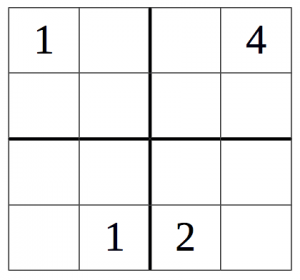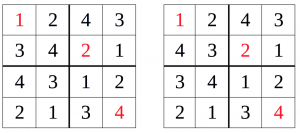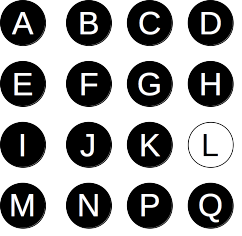## ________________

Coach Newton explains: “If you see something like 354 in base eight, how do you figure out what number it is? You use the powers of eight (1, 8, 64, 512, …) just the way you would ordinarily use 1, 10, 100, 1000, and so on, for the place values of a numeral. So you would get 3×64 + 5×8 + 4×1. The same sort of thing works to interpret 354 in any base b greater than 5: the value would be 3×b² + 5×b + 4×1. Here are some puzzles about different number bases.”

## ________________### Frequent Figures

Given a positive whole number n, say that a base b < n is a “copycat” base for n if the following two conditions hold: (a) only one digit, d, occurs in the base b representation of n, and (b) that digit occurs exactly d times.

What is the smallest number with two different copycat bases?

### Doubled Digit

If you raise seven to the eleventh power, you get a number which, when written in base nine, has ten digits. All of the nine possible digits from zero to eight occur in that ten-digit number. (The digit “9” cannot appear in a base-nine number.) Since it has ten digits in all, exactly one of the digits repeats somewhere in the number.

Which digit appears twice in the base nine representation of seven to the eleventh power?

## Solutions to week 73Small Sudoku. First, note that the diagram at left has only four clues, and it has a unique solution; in fact, if you fill in the cells in the order indicated by the letters in the solution to the right (a-b-c-d-…), there is only one option for each cell in turn.

What if a 4-by-4 Sudoku has only three clues? First, notice that you can take any Sudoku solution and perform any of the following operations on it to get another Sudoku solution: swap the top two rows with the bottom two rows; swap the left two columns with the right two columns; swap the first and second rows or the third and fourth rows; swap the first and second columns or the third and fourth columns; reflect the puzzle about either diagonal; or swap the positions of all occurrences of any one digit and any other digit. We reach three conclusions from these operations: First, there must be clues in two diagonally opposite 2-by-2 sub-boxes, because otherwise we could modify the solution without changing any clues. Second, all three clues must be different digits (otherwise we could just swap the two digits that did not show up in the clues). Third, we may as well assume (by transforming both the clues and the solution) that the clues include a “1” in the upper left corner and a “4” in the lower right corner, with a “2” clue somewhere else in the grid.

Now consider the two diagrams below to see that the grid has two different solutions: If the additional clue of a “2” occurs in one of the colored squares, then in each diagram, fill in whichever color the “2” appears in with all 2s, and the other color with 3s. That produces two different solutions. If the “2” appears in a square that has a “1” or a “4” in it, try reflecting both diagrams about the main diagonal that goes from the upper left to the bottom right. If now the “2” is in a colored square, follow the same procedure with the reflected diagrams to get two solutions.Finally, if the “2” is still not in a colored square, then it must (after possibly reflecting about the main diagonal) be in the second row and third column. But that diagram has two solutions, as you can see below. Hence it is impossible to uniquely pin down every entry with just three clues, so you need four clues at a minimum.Puny Pegs.No jumps are possible at all on a 1-by-1 or 2-by-2 board, so those sizes can’t work. On a 3-by-3 board, the corner pegs can jump, but nothing can jump them. So every move leaves at least three corner pegs (since there are at least three to start), so it can’t be possible to reduce to one peg, so that size doesn’t work either.

However, starting with the pictured 4-by-4 configuration, it is possible to reduce to a single peg with the following sequence of jumps: J→L B→J I→K A→I M→E H→F E→G L→J N→F C→K P→G F→H D→L Q→H. So the smallest board that can be solved from one space to one peg is a 4-by-4 board.

## Recent Weeks

Links to all of the puzzles and solutions are on the Complete Varsity Math page.

Come back next week for answers and more puzzles.

[asciimathsf]